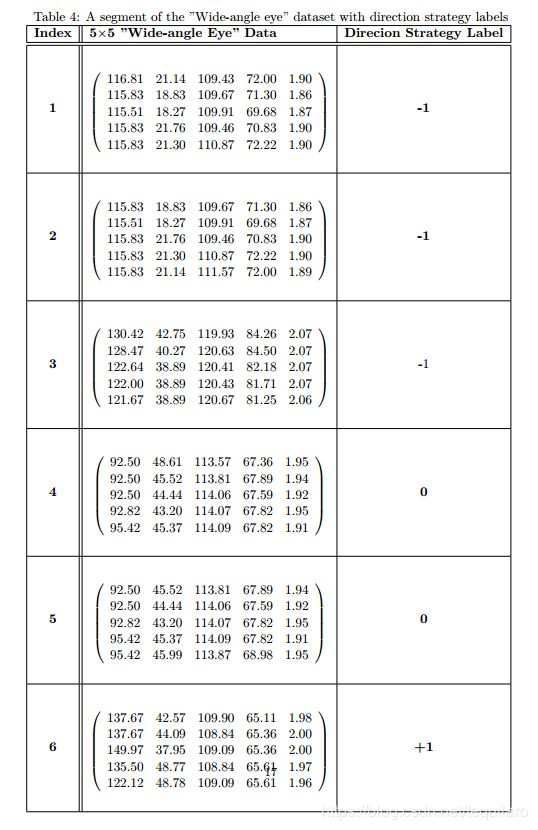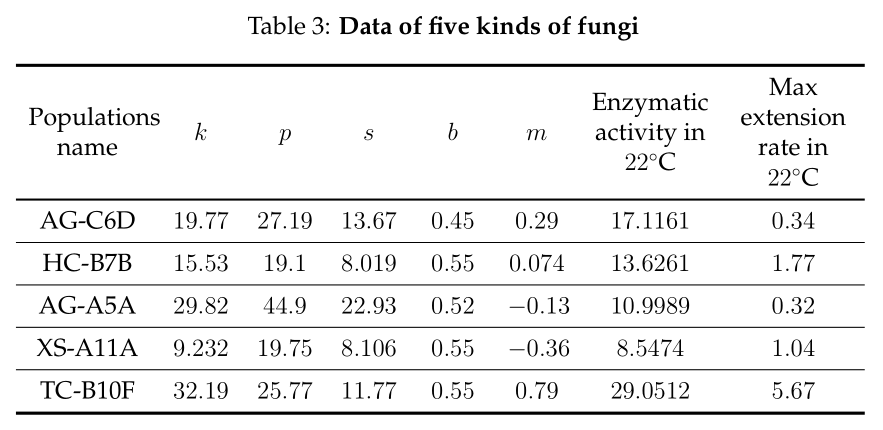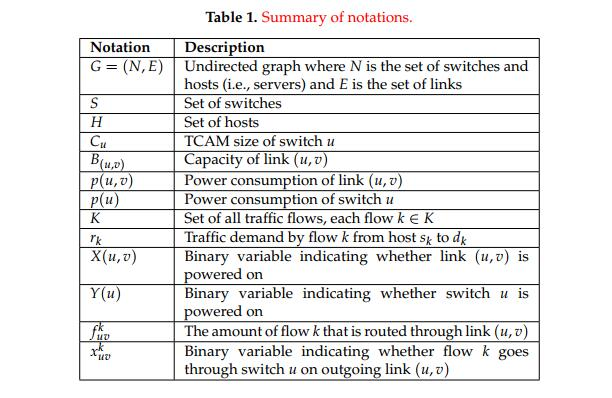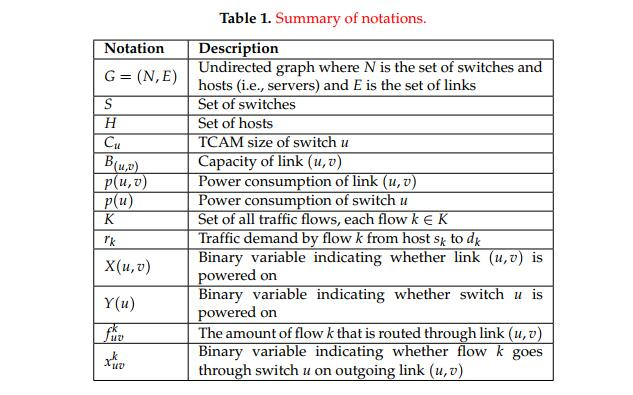• Latex表格内容垂直居中Latex内容的编写经常会涉及到Excel表格的编写，但是直接按照正常的方式编写那么就会使得文字不能居中显示，所以下述给出Excel文字垂直居中的方法。首先记得导入array包。\usepackage{array}...
Latex表格内容垂直居中Latex内容的编写经常会涉及到Excel表格的编写，但是直接按照正常的方式编写那么就会使得文字不能居中显示，所以下述给出Excel文字垂直居中的方法。首先记得导入array包。\usepackage{array}这样才能使用m{7cm}的指令，是使得Excel中的内容垂直居中显示。我的代码如下，数字请忽略。\begin{table*}\centering\caption{A segment of the "Wide-angle eye" dataset with direction strategy labels}\begin{tabular}{|c|| m{7cm} |c|}\hline\bfseries Index & \bfseries 5$\times$5 "Wide-angle Eye" Data & \bfseries Direcion Strategy Label\\\hline\hline\bfseries 1 &\begin{equation}\left(\begin{array}{ccccc}116.81 & 21.14 & 109.43 & 72.00 & 1.90\nonumber\\115.83 & 18.83 & 109.67 & 71.30 & 1.86\nonumber\\115.51 & 18.27 & 109.91 & 69.68 & 1.87\nonumber\\115.83 & 21.76 & 109.46 & 70.83 & 1.90\nonumber\\115.83 & 21.30 & 110.87 & 72.22 & 1.90\nonumber\\\end{array}\right)\end{equation}& \bfseries-1\\\hline\bfseries 2 &\begin{equation}\left(\begin{array}{ccccc}115.83 & 18.83 & 109.67 & 71.30 & 1.86\nonumber\\115.51 & 18.27 & 109.91 & 69.68 & 1.87\nonumber\\115.83 & 21.76 & 109.46 & 70.83 & 1.90\nonumber\\115.83 & 21.30 & 110.87 & 72.22 & 1.90\nonumber\\115.83 & 21.14 & 111.57 & 72.00 & 1.89\nonumber\\\end{array}\right)\end{equation}& \bfseries-1\\\hline\bfseries 3 &\begin{equation}\left(\begin{array}{ccccc}130.42 & 42.75 & 119.93 & 84.26 & 2.07\nonumber\\128.47 & 40.27 & 120.63 & 84.50 & 2.07\nonumber\\122.64 & 38.89 & 120.41 & 82.18 & 2.07\nonumber\\122.00 & 38.89 & 120.43 & 81.71 & 2.07\nonumber\\121.67 & 38.89 & 120.67 & 81.25 & 2.06\nonumber\\\end{array}\right)\end{equation}& -1\\\hline\bfseries 4 &\begin{equation}\left(\begin{array}{ccccc}92.50 & 48.61 & 113.57 & 67.36 & 1.95\nonumber\\92.50 & 45.52 & 113.81 & 67.89 & 1.94\nonumber\\92.50 & 44.44 & 114.06 & 67.59 & 1.92\nonumber\\92.82 & 43.20 & 114.07 & 67.82 & 1.95\nonumber\\95.42 & 45.37 & 114.09 & 67.82 & 1.91\nonumber\\\end{array}\right)\end{equation}& \bfseries 0\\\hline\bfseries 5 &\begin{equation}\left(\begin{array}{ccccc}92.50 & 45.52 & 113.81 & 67.89 & 1.94\nonumber\\92.50 & 44.44 & 114.06 & 67.59 & 1.92\nonumber\\92.82 & 43.20 & 114.07 & 67.82 & 1.95\nonumber\\95.42 & 45.37 & 114.09 & 67.82 & 1.91\nonumber\\95.42 & 45.99 & 113.87 & 68.98 & 1.95\nonumber\\\end{array}\right)\end{equation}& \bfseries 0\\\hline\bfseries 6 &\begin{equation}\left(\begin{array}{ccccc}137.67 & 42.57 & 109.90 & 65.11 & 1.98\nonumber\\137.67 & 44.09 & 108.84 & 65.36 & 2.00\nonumber\\149.97 & 37.95 & 109.09 & 65.36 & 2.00\nonumber\\135.50 & 48.77 & 108.84 & 65.61 & 1.97\nonumber\\122.12 & 48.78 & 109.09 & 65.61 & 1.96\nonumber\\\end{array}\right)\end{equation}& \bfseries +1\\\hline\end{tabular}\label{tbl:table4}\end{table*}最后latex编译得到的图片如图所示。这样就可以编辑出一个很棒的Excel表格了。谢谢大家。如果还有什么关于Latex编辑的问题可以互相讨论。
展开全文• 可能由于设置latex表格列宽过小导致的文字不居中垂直和水平居中） 解决办法：采用如下改动：m代表middle，|为表格竖线，2.5cm代表列宽，<{centering}为居中. \begin{tabular}{m{1cm}<{\centering}|m{1cm}<...
Latex 表格文字居中（垂直和水平居中）
可能由于设置latex表格列宽过小导致的文字不居中（垂直和水平居中）
解决办法：采用如下改动：m代表middle，|为表格竖线，2.5cm代表列宽，<{centering}为居中.
\begin{tabular}{m{1cm}<{\centering}|m{1cm}<{\centering}|m{1cm}<{\centering}|m{1cm}<{\centering}}
需要导入array包： \usepackage{array}
参考这 https://www.freesion.com/article/6635554950/
若仍然不行：内容缩写吧！abbr.


展开全文• m表示垂直居中，2cm表示该单元格水平宽度2厘米。 那水平居中怎么办呢？ 在后面加<{\centering} 注意有多少列，就要加多少个参数，每一列的参数可以不同。 用如下代码，注意\begin{tabular}后面的参数部分： %注意...
网上的方法杂七杂八，有些用multicolumn的，很麻烦，后来参考了很多地方，总结除了自己的经验。
首先最前面加入包：
\usepackage{array}

然后在\begin{tabular}后面加参数m{2cm}
m表示垂直居中，2cm表示该单元格水平宽度2厘米。
那水平居中怎么办呢？
在后面加<{\centering}
注意有多少列，就要加多少个参数，每一列的参数可以不同。
用如下代码，注意\begin{tabular}后面的参数部分：
%注意最前面加包\usepackage{array}

\begin{table}[H]
\caption{\centering\textbf{Data of five kinds of fungi}}%标题
\label{tab:0003}
\centering%把表居中
<{\centering}p{1cm}<{\centering}p{2cm}<{\centering}p{2cm}<{\centering}
\begin{tabular}{m{2cm}<{\centering}m{1cm}<{\centering}m{1cm}<{\centering}m{1cm}<{\centering}m{1cm}<{\centering}m{1cm}<{\centering}m{2cm}<{\centering}m{2cm}<{\centering}}
\toprule[1.5pt]%第一道横线
Populations name & $k$ & $p$ & $s$ & $b$ & $m$ & Enzymatic activity in $22^\circ$C & Max extension rate in $22^\circ$C\\
\midrule[1pt]%第二道横线
AG-C6D	& $19.77$ & $27.19$ & $13.67$ & $0.45$ & $0.29$ & $17.1161$ & $0.34$ \\
\midrule[0.5pt]
HC-B7B & $15.53$ & $19.1$& $8.019$& $0.55$& $0.074$& $13.6261$& $1.77$ \\
\midrule[0.5pt]
AG-A5A	& $29.82$ & $44.9$& $22.93$& $0.52$& $-0.13$& $10.9989$& $0.32$ \\
\midrule[0.5pt]
XS-A11A	& $9.232$ & $19.75$& $8.106$& $0.55$& $-0.36$& $8.5474$& $1.04$ \\
\midrule[0.5pt]
TC-B10F & $32.19$ & $25.77$& $11.77$& $0.55$& $0.79$& $29.0512$& $5.67$ \\

\bottomrule[1.5pt]%第三道横线
\end{tabular}
\label{table:data}
\end{table}

效果图：展开全文• 最近在用Latex绘制表格，遇到了两个问题，记录一下。 1、一个是某一单元格内容过长，换行的问题 关键代码：\begin{tabular}{|l|p{7.3cm}|} 网上查找说可以用p{}来控制列的宽度，如p{7.3cm}表示控制表格的最大宽度...
最近在用Latex绘制表格，遇到了两个问题，记录一下。

1、一个是某一单元格内容过长，换行的问题

关键代码：\begin{tabular}{|l|p{7.3cm}|}

网上查找说可以用p{}来控制列的宽度，如p{7.3cm}表示控制表格的最大宽度为7.3cm，超过则换行。

上代码：

\begin{table}[H]
\centering
\caption{\textcolor{red}{Summary of notations.}}
\label{T1}
\begin{tabular}{|l|p{7.3cm}|}
\hline
{\bf \small Notation}& {\bf\small Description}\\
\hline
$G = (N, E)$     & Undirected graph where $N$ is the set of switches and hosts (i.e., servers) and $E$ is the set of links\\
\hline
$S$      &    Set of switches\\
\hline
$H$      &   Set of hosts\\
\hline
$C_{u}$     &  TCAM size of switch $u$\\
\hline
$B_{(u,v)}$     & Capacity of link $(u,v)$\\
\hline
$p(u,v)$      &  Power consumption of link $(u,v)$\\
\hline
$p(u)$      &   Power consumption of switch $u$\\
\hline
$K$     &    Set of all traffic flows, each flow $k\in K$\\
\hline
$r_{k}$      &  Traffic demand by flow $k$ from host $s_{k}$ to $d_{k}$\\
\hline
$X(u,v)$      &  Binary variable indicating whether link $(u,v)$ is powered on\\
\hline
$Y(u)$      &   Binary variable indicating whether switch $u$ is powered on\\
\hline
$f_{uv}^{k}$  &  The amount of flow $k$ that is routed through link $(u,v)$\\
\hline
$x_{uv}^{k}$  &  Binary variable indicating whether flow $k$ goes through switch $u$ on outgoing link $(u,v)$\\
\hline
\end{tabular}
\end{table}

效果图换行实现了，再看第一列，符号没有居中对齐。

2、左对齐垂直居中的问题

我们只要把上述关键代码改为：

关键代码：\begin{tabular}{|l|m{7.3cm}|}

即p改为m，应该m是一个居中的意思。

效果如下图展开全文• 下面的例子提供了「强行让单元格内容纵向居中」的方案，仅作为一种技术实现，不推荐实际使用(因为这种效果打破了排版的惯例)。\documentclass[a4paper]{ctexart}\usepackage{amsmath}\usepackage{amssymb}\...
• Latex表格内容垂直居中 Latex内容的编写经常会涉及到Excel表格的编写，但是直接按照正常的方式编写那么就会使得文字不能居中显示，所以下述给出Excel文字垂直居中的方法。 首先记得导入array包。\usepackage{array} ...
• 试了一下，LaTeX表格中的单元格在单行的情况下在竖直方向上应该是垂直居中的，所以我猜测您的问题应该是在限定了列宽之后由于自动换行导致的其他列无法做到在竖直上居中，解决方法是使用multicolumn命令，以下为简单...
• 在撰写论文时，常常使用table制作表格，不过存在一个问题：单元格内文本顶着上格线，不好看。解决方案有两种思路。 其一：利用multicolumn命令 制作四行四列表格的代码如下： \begin{center} \begin{tabular}{ | ...
• 我相信许多人在使用Latex制作表格的时候，都遇到过表格中的文字不能垂直居中的问题。 本例子是在表格中插入图片作为例子的： \begin{figure} \centering \begin{tabular}{cccc} % 表格中的PXX代表图片 \...经验分享
• 后续讨论tabular默认让单元格里的内容靠上，快顶线了很不美观默认的行为是，行高由行内最高单元格决定，列标 lrc，纵向靠下对齐列标 mpb（其中mb 需加载宏包 array），在当前行的行高是被 mpb 撑高时，纵向居中对齐...
• 利用Latex在线表格绘制表格以及多行合并垂直居中 之前用latex绘制表格时都是直接写程序，但是对于复杂的表格，自己设计程序以及书写程序时非常麻烦，在用latex写论文时表格的绘制就花费了非常多的时间，要是绘制的...
• 如何同时让表格同一行一个单元格的文字能垂直居中？比如说文字超长超出页面范围需要分行显示。 ~~~newcommand{tabincell}{begin{tabular}{@{}#1@{}}#2end{tabular}}%放在导言区%然后使用 tabincell{c}{} 就可以在...
• latex表格行高、文本居中 m{7cm}垂直居中 加array宏包
• 1、在导言区添加宏包：\usepackage{makecell} ...*[c]水平 + 垂直居中，*[l]垂直居中 + 水平左居中，*[r]垂直居中 + 水平右居中。 注意：makecell里文本内容想换行，就用 \\ 分隔即可；该方法适用于指定换行的地方 ...windows
• 1、在导言区添加宏包： \usepackage{makecell} 2、环境：tabular 命令： \makecell[居中情况]{第1行内容 \\ 第2行内容 \\ 第3行...[c]水平 + 垂直居中，[l]垂直居中 + 水平左居中，*[r]垂直居中 + 水平右居中。 ...
• Latex 表格单元格内换行问题：如何同时让表格同一行一个单元格的文字能垂直居中？比如说文字超长超出页面范围需要分行显示答：（来源于smth）方案一：/newcommand{/tabincell}{/begin{tabular}{@{}#1@{}}#2/end{...c
• 首先导入必须的包 \usepackage{array} \begin{tabular}{|m{3.5cm}<{\centering}|m{2cm}<...其实3.5cm表示每一列的列宽度，m表示垂直居中，可选项为b（置底），p（置顶）。主要在于表头设置，以及必...
• 问题：如何同时让表格同一行一个单元格的文字能垂直居中？比如说文字超长超出页面范围需要分行显示答：（来源于smth）方案一：/newcommand{/tabincell}{/begin{tabular}{@{}#1@{}}#2/end{tabular}}%然后使用&/...c
• 问题：如何同时让表格同一行一个单元格的文字能垂直居中？比如说文字超长超出页面范围需要分行显示 答：（来源于smth） 方案一： \newcommand{\tabincell}{\begin{tabular}{@{}#1@{}}#2\end{tabular}} ...
• \documentclass{ctexart}%表格使用tabular环境，【垂直对齐方式】{列个是的说明}% l-表示左对齐，c表示居中对齐，r表示右对齐，,% 设置每一列数据对齐方式，，还可以设置竖线,\hline是横线%用&表示不同的列%两个...
• 表示表格的分隔，\\表示下一行表格，c表示居中对其，(lr)分别表示左右对齐。例如我们在当前位置插入表格(2x3),如果要给表格左右加上框则可以|ccc|,垂直方向上加上横线可以使用:\hline \begin{tabular}{ccc} 第一...tabular
• 1. Latex 表格单元格内换行问题：如何同时让表格同一行一个单元格的文字能垂直居中？比如说文字超长超出页面范围需要分行显示答：（来源于smth）方案一：/newcommand{/tabincell}{/begin{tabular}{@{}#1@{}}#2/...html c string 语言